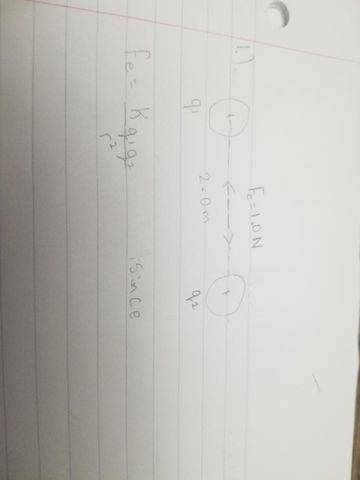# Find the charge of the sphere (q2)

• NotInMrPutmansClass

#### NotInMrPutmansClass

Homework Statement
Two small spheres (q1& q2) have different charges that add up to 5.0x10^-5 C. If they repel each other with a force of Fe = 1.0 N at a distance of 2.0 m apart, what is the charge on q2?
Relevant Equations
Electric force formula

Fe= (k*q1*q2)/r^2

Electric Field Formula

E=(k*q1)/r^2

where k is a constant 9x10^9 N*m^2/kg^2
I drew a diagram using all of the informationhowever, I am stuck and not to sure how to get one of the charges

Try writing out the equation that says the charges add up to…

See if that gives you enough info.